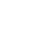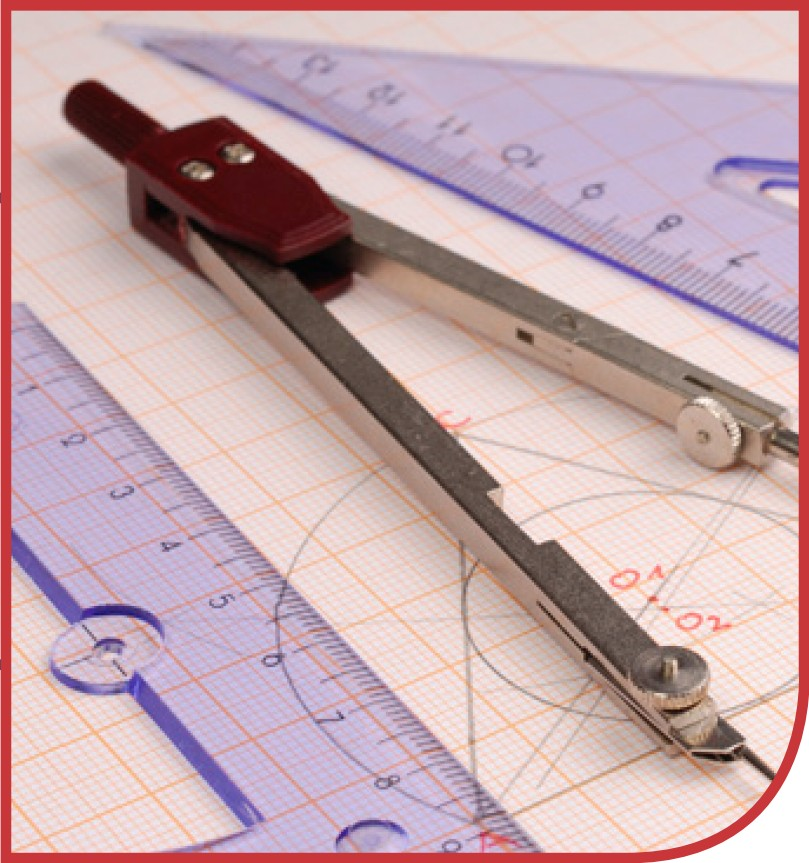Graduate division of National Paralegal College### MAT-103COLLEGE GEOMETRY 3 Credits

This course is a part of:
Associate's Degree Program
Bachelor's Degree Program

This course is designed to introduce the student to the basic principles of geometry necessary for further college level mathematics. These principles include properties of geometric shapes and measurements, calculating the dimensions, including one, two and three dimensional properties of geometric figures. The course also discusses concepts such as similarity and congruency and geometric proofs establishing relationships between figures. The course continues with characteristics of triangles, quadrilaterals, higher order polygons, circles, three dimensional figures and the properties of the sides and angles appurtenant to these figures. Finally, the course finished with graphing and coordinate geometry.

## Course Objectives

By the end of the course, the student will be able to:

• Solve real life problems using geometry
• Identify geometric shapes and characteristics of angles, lines and shapes
• Determine whether a geometric figure is congruent and/or similar to another given figure and explain the reasons for such conclusion
• Calculate the perimeter, area and volume of a variety of geometric figures
• Apply the rules of geometry of a circle to circles themselves and properties of lines and angles that run through one or more points on the circle’s circumference
• Plot points, lines and geometric figures on a graph and use coordinate geometry rules to identify properties of these points, lines and figures.